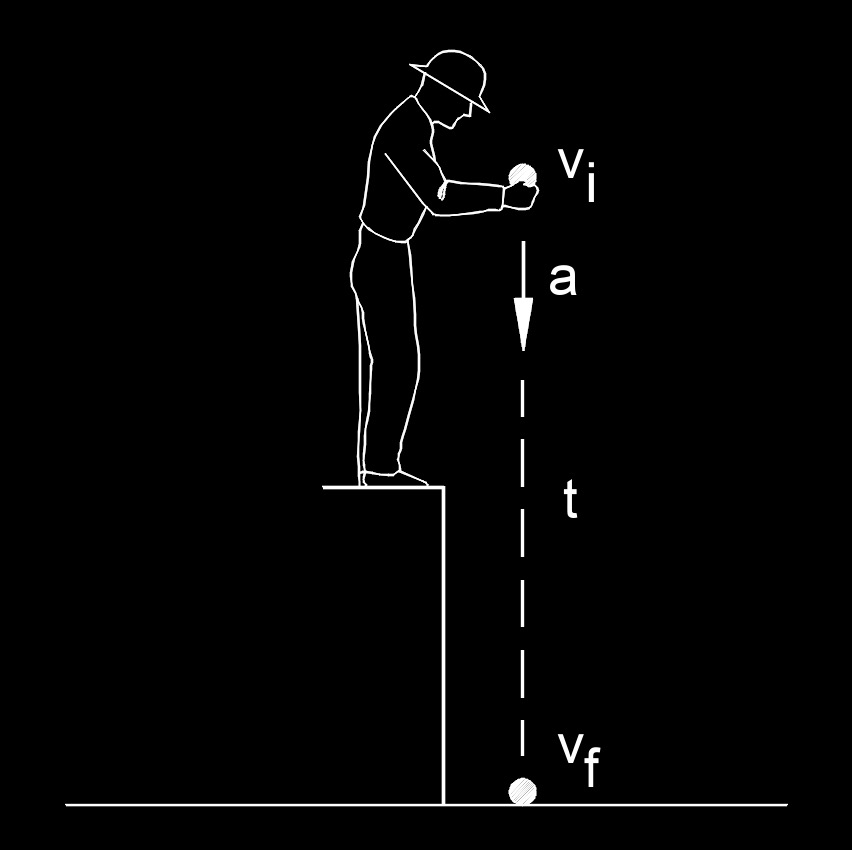# Final Velocity

Written by Jerry Ratzlaff on . Posted in Classical MechanicsFinal velocity, abbreviated as $$v_f$$, is the ending point of motion.

## Final Velocity formulas

 $$\large{ v_f = v_i + a \; t }$$ $$\large{ v_f = 2 \; \bar {v} - v_i }$$ $$\large{ v_f = \alpha_v \; v_i \; \left( T_f \;- \; T_i \right) + v_i }$$ (volumetric thermal expansion coefficient)

### Where:

$$\large{ v_f }$$ = final velocity

$$\large{ a }$$ = acceleration

$$\large{ T_f }$$ = final temperature

$$\large{ T_i }$$ = initial temperature

$$\large{ t }$$ = time

$$\large{ \bar {v} }$$ = average velocity

$$\large{ v_i }$$ = initial velocity

$$\large{ \alpha_v }$$  (Greek symbol alpha) = volumetric thermal expansion coefficient